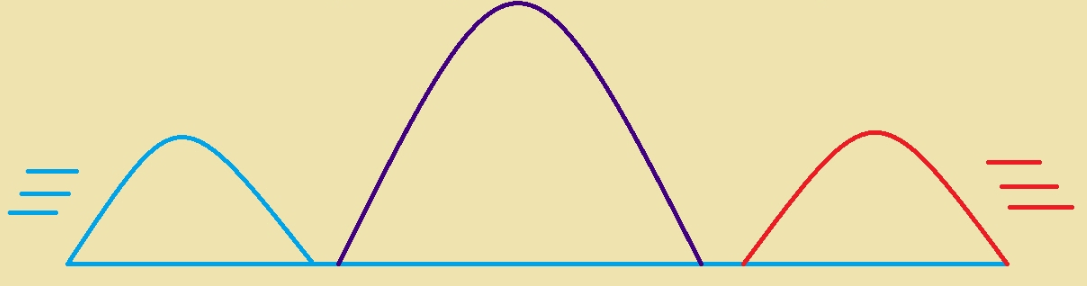# Principle of Superposition of Waves

The presence of the waves around us works to channelize the various phenomenon. Imagine if one is sailing in the boat, or just hear the siren of the ship. In these cases, one will be able to directly receive the sound wave from the siren of the ship, as well as the sound wave that is reflected by the seawater. For understanding the concept of the superposition of the waves, the depth in knowledge about the superposition theorem is required.## Introduction to Superposition of Waves

By taking the example of the string wave for defining the principle of the superposition of the waves that is exactly based on the superposition theorem, superposition of the waves can be expressed. According to this net displacement of any of the components, the string in the given time is equal to the algebraic total of the displacement that is caused due to each of the waves. Hence the method of adding up to the individual waveforms for evaluating the net waveform is termed as the principle of superposition.The principle of the superposition can be expressed by affirming that the overlapping waves are added algebraically, for creating the constant value. Based on the principle of superposition, the motion, or the travel of the waves is not affected hampered by the overlapping waves. Therefore, the wave function labels the disturbance in the medium can be denoted as follows.So, the superposition of the waves can lead to the statement of the following three effects.

• Whenever, the two waves have the same frequency, traveling with the speed along with the same direction in the specified medium, then they superpose and create the effect of that is termed as the interference of the waves.
• In the situation when the two waves have a similar frequency, which is moving at the same speed, along the opposite direction in the specified medium, then they superpose for producing the stationary waves.
• Finally, when the two different waves have slightly varying frequencies and they are traveling with the same speed along the same direction, in the specified medium, they superpose for producing the beats.

## Types of Superposition of Waves

Depending on the phase differences, in the superimposing waves, then the interference is divided into two parts such as constructive interference and destructive interference. When the two waves superimpose with each other in the same phase then the resultant amplitude is equal to the sum of the amplitude of the different waves that result in the maximum light intensity and it is known as the constructive interference. But if two waves superimpose each other in the opposite direction then the resultant amplitude is equal to the difference, in the amplitude of the waves causing the production of minimum light intensity and it is known as the destructive interference.